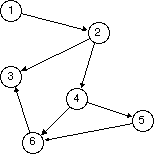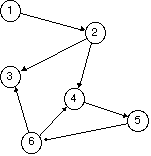Welcome to Lesson 15!Learning ObjectivesWhat is a Graph?What are some real world applications of Graphs?What are two ways to represent a Graph?What is a vertex and what is an edge?What is an origin and what is a terminus or destination?Can a vertex be connected to itself by an edge?What is the degree of a vertex?What does it mean to be an adjacent vertex?How is a directed Graph different from an undirected Graph?What is a path?What is a cycle?What is a parallel edge?What is a DAG?What is Tree?How would you represent the Graph ADT as a data structure?1. Introduction to GraphsToday we are going to learn about the Graph ADTThese graphs are not the same as the graphs that you learned about in Algebra and Calculus.Instead, there is a whole field of study known as Graph Theory which deals with the pairwise relationship between objects.In other words, we will be looking at the connected pairs of objects to see how these objects relate to each other.1.1. Applications of Graph TheoryModeling transportation networks, such as highways and flight paths.Representing relationships between components in electronic circuitsComputer networks: LAN, Internet, WebRepresenting dependency of tables in databasesWe will watch a video about one Graph application at the end of class.1.2. Graph DefinitionWe can define a graph as a set (or collection) of vertices (the nexus points) and edges (the lines connecting the vertices together). G= {V,E}For example, take a look at the following graph:Image source.Using set theory, we can define this Graph like so:V(G) = { 1, 2, 3, 4, 5, 6, 7}E(G) = {15, 17, 57, 45, 46, 67, 27, 26, 23, 34}V(G) is called the vertex set. It is an un-ordered list of all the vertices in the Graph.E(G) is called the edge set. It is an un-ordered list of all the edges in the Graph.These two components are what define the graph.There are two other ways to define graphs, not involving setsWe will learn about one of these at the end of class today.1.3. VerticesA vertex (plural vertices) or node is the fundamental unit of which graphs are formed.At its most basic, a graph can be defined as a single vertex with no edges.The below is considered a valid graph (no it's not an M&M), although no edges are present:In addition, vertices also have a degree. The degree of a vertex is the number of edges that are coming from it (incident upon it).The degree of the above vertex is 0.What is the degree of vertex 6 above?Below is an image of a graph which is labeled with the degrees of each vertex:Image source.1.4. EdgesAn edge is defined by the vertices between it, as a pair (u, v).u and v are both vertices in the graph, with the edge lying between them.The vertex u is called the origin, the vertex that the edge is coming from.The vertex v is called the destination or terminus, the vertex that the edge is coming to.The above is an example of a directed edgeA directed edge is a pair of ordered vertices.In other words, u is always the origin and v is always the destination.When we draw a directed edge, we represent it with an arrow. The arrow is coming from the origin and pointing to the destination vertex.Below is an example of an undirected edge.Note that there is no arrow connecting the two. An undirected edge is an unordered pair of vertices (u,v), where either u or v could be the origin.1.5. Directed and Undirected GraphsGraphs themselves can be directed or undirected.Directed graph: all edges are directed.The following is an example of a directed graph:Image source.Undirected graph: all edges are undirected.Image source.1.6. AdjacencyWhen an edge connects two vertices, the vertices are said to be adjacent to each other.Any edge that connects two adjacent vertices is said to be incident on those vertices.Two edges are said to be adjacent if they share a common vertex.1.7. Reflection:In the undirected graph above, which vertices are adjacent?Give two examples of adjacent edges.1.8. Self Loops and Parallel EdgesA self loop occurs when a vertex is adjacent to itself.For example, vertex 4 in the graph below contains a self loop:Image source.Parallel edges occur when two vertices are adjacent to each other - twice. In other words, there is a loop from one vertex to another.Parallel edges can be seen in the graph below between vertices 1 and 8 and also between vertices 6 and 8.Can you also spot the self loops?Image source.1.9. Connected and Disconnected GraphsLook at the following image below. Does it meet our definition of a graph?Image sourceIt turns out the answer is yes.The above is called a disconnected graph.A disconnected graph is one in which there is no simple path to all the vertices in the graph.We can define a path as any route through a graph from vertex to vertex along edges, where no vertex along this route is repeated.For example, there are two paths from vertex b to vertex a above: b-c-d-a and b-a.However, there is no path from g to a or from h to d. Thus, our graph above is disconnected.A connected graph is one in which there is a path to every vertex in the graph.For example, the below graph is connected:Notice that there is a path from every vertex to every vertex in the above graph.A directed graph is called weakly connected if replacing all of its directed edges with undirected edges produces a connected (undirected) graph.For example, the below graph is weakly connected:image sourceIf a graph is disconnected, it is considered to be composed of connected components.For example, the below graph contains 2 connected components:1.10. Cyclic and Acyclic GraphsA cyclic graph is one which contains a cycle.A cycle consists of a sequence of vertices starting and ending at the same vertex, with each two consecutive vertices in the sequence adjacent to each other in the graph. Inside a cycle, there should be no repetition of vertices, except the first vertex, which is also the last vertex.In the below graph, H-D-G-H is a cycle.Can you see any others?In a directed graph, a cycle must follow the edges from origin to destination vertex.For example, the following graph contains the cycle B-C-E-D-B:Image source.However, the below graph is considered to be acyclic, meaning that it contains no cycles.Image source.1.11. Directed Acyclic Graphs (DAGs)A directed acyclic graph, a.k.a. a DAG, is a special type of graph.Among other applications, DAGs are useful in scheduling, when there are a collection of tasks that must be ordered into a sequence, but are subject to constraints that certain tasks must be performed earlier than others.Given the images below, which one is a DAG and which one is not?1.12. Trees RevisitedA tree is a graph that is both connected and acyclic.Below is an example of a tree.Image source.In graph theory, we usually consider all trees to be undirected. However, computer science, unlike applied math, allows for trees with directed edges, thus complicating our definition.A directed tree is a directed graph which would be a tree if the directions on the edges were ignored.1.13. Reflection ActivityDecide whether the following Graphs are: connected or disconnectedcyclic or acyclicdirected or undirectedGraph A:Graph B:Image source.Graph C:Graph D:Image source.2. Applications of Graph TheoryVideo: Graph Theory and Maps3. Writing a Graph Data StructureLet's start to think programmatically about how we will represent a Graph as a data structure.As we know, a Graph is simply a set of vertices and edges.The vertices can be written as a vertex set and the edges as an edge set.We saw an example an example of a vertex and edge set at the beginning of class.Vertex and Edge Sets for Graph G:        V(G) = { 1, 2, 3, 4, 5, 6, 7}           E(G) = {15, 17, 57, 45, 46, 67, 27, 26, 23, 34}3.1. Adjacency ListsIn addition to using sets, there is another way to represent a Graph using what are called adjacency lists.An adjacency list representation of a graph is a collection of unordered lists, one for each vertex in the graph. Each list contains the set of adjacent vertices to that vertex.For example, I could represent the above Graph G using an adjacency list like so:1: 5, 72: 3, 6, 73: 2, 4, 64: 3, 5, 65: 1, 4, 76: 2, 3, 4, 77: 1, 2, 5, 63.2. Reflection ActivityWrite the adjacency list for the following Graphs:How is the adjacency list different when the Graph is directed vs undirected?Graph G1:Image source.Graph G2:3.3. Adjacency Lists and the Graph Data StructureUsing the adjacency list representation of a Graph makes it easy to represent a Graph in code.We can use an array (or ArrayList) of linked lists.Each index of the array represents one vertex in our Graph.The array at this location represents the adjacency list for that Graph.We can see this representation as the depiction below:Image source.Wrap UpAnswer the review questions for this lesson on Canvas~Have a Great Day!~